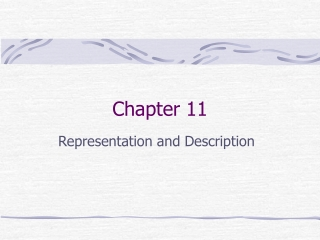Download PresentationChapter 11

Loading in 2 Seconds...

# Chapter 11

Download Presentation## Chapter 11

- - - - - - - - - - - - - - - - - - - - - - - - - - - E N D - - - - - - - - - - - - - - - - - - - - - - - - - - -
##### Presentation Transcript

1. Chapter 11 Representation and Description

2. Preview • Representing a region involves two choices: • In terms of its external characteristics (its boundary) • In terms of its internal characteristics (the pixels comprising the region) • Describe the boundary by features such as length, orientation, number of concavities. • Descriptors should be as insensitive as possible to variations in size, translation and rotation.

3. Representation: Chain Code • Represent a boundary by a connected sequence of straight-line segment of specified length and direction. • Based on 4- or 8-connectivity

4. Chain Code

5. Polygonal Approximations • Minimum perimeter polygons • Merging techniques • Splitting techniques

6. Signatures • 1-D functional representation of a boundary.

7. Boundary Segments • Convex hulls, convex deficiency

8. Skeletons • Medial axis transform (MAT) • Thinning process

9. Boundary Descriptors • Simple descriptors: length, diameter, major/minor axis, ratio of major to minor axis (eccentricity), curvature. • Shape numbers

10. Fourier Descriptors • (x0,y0),(x1,y1,),…,(xK-1,yK-1) • Viewed a series of complex numbers s(k)=x(k)+jy(k) • Take Fourier transform of s(k) and keep only P terms. (P < K)

11. Illustration

12. Statistical Moments • The nth moment of v about its mean is defined as:

13. Regional Descriptors • Simple descriptors: area, perimeter, compactness, mean, median, max, min. • Topological descriptor: Euler number E=C-H. • Texture • Moments of 2-D functions

14. More descriptors • PCA • Relational descriptors